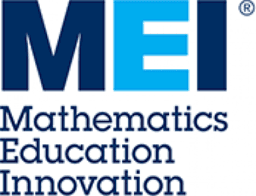# Visualizing Functions with Mapping Diagrams: MEI 2019

Visualizing Functions with Mapping Diagrams: from Linear and Quadratic to Exponential and Trigonometric Using recent work with GeoGebra on mapping diagrams, an alternative to graphs, I will provide visualizations that can help students understand functions. Participants will use mapping diagrams to visualize the solution of equations based on linear and quadratic functions. Diagrams for simple polynomial as well as exponential and trigonometric functions will be considered as well as the relation of quadratic and cubic equations to their complex number solutions. Links: https://flashman.neocities.org/Presentations/MEI/MEI.MD.LINKS.html